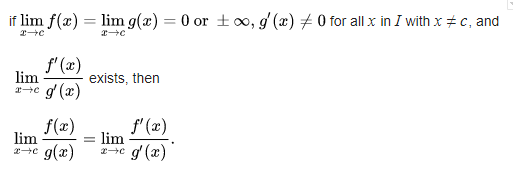# L'Hospital's Rule

In Calculus, the most important rule is L’ Hospital’s Rule (L’Hôpital’s rule). This rule uses the derivatives to evaluate the limits which involve the indeterminate forms. In this article, we are going to discuss the formula and proof for the L’Hospital’s rule along with examples.

## What is L’Hospital’s Rule?

L’Hospital’s rule is a general method of evaluating indeterminate forms such as 0/0 or ∞/∞. To evaluate the limits of indeterminate forms for the derivatives in calculus, L’Hospital’s rule is used. L Hospital rule can be applied more than once. You can apply this rule still it holds any indefinite form every time after its applications. If the problem is out of the indeterminate forms, you can’t be able to apply L’Hospital’s Rule.

## L’Hospital’s Rule Formula

L’hospital’s rule states thatNote: The limit of the quotient of function is equivalent to the limit of the quotient of their derivatives, given that the provided conditions are satisfied.

## L’Hospital’s Rule proof

By the use of Extended Mean Value Theorem or Cauchy’s Mean Value Theorem, the L’Hospital’s rule can be proved.

If f and g are two continuous functions on the interval [a, b] and differentiable on the interval (a, b), the

f’(c)/g’(c) = [f(b)-f(a)]/[g(b)-g(a)] such that c belongs to (a, b).

Assume that the two functions f and g are defined on the interval (c, b) in such a way that f(x)→0 and g(x)→0, as x→c+.

But we have f’(c) / g’(c) tends to finite limits. The functions f and g are differentiable, and f’(x) and g’(x) exists on the set [ c, c+k], and also f’ and g’ are continuous on the interval [c, c+k] provided with the conditions f(c)= g(c) = 0 and g’(c) ≠ 0 on the interval [c, c+k].

By Cauchy Mean Value Theorem states that there exists ck∈ (c, c+k), such that

f’(ck)/g’(ck) = [f(c+k)-f(c)]/[g(c+k)-g(c)] = f(c+k)/g(c+k)

Now, k→0+,

$$\begin{array}{l}\lim_{k\rightarrow 0^{+}}\frac{f'(c_{k})}{g'(c_{k})}=\lim_{x\rightarrow c^{+}}\frac{f'(x)}{g'(x)}\end{array}$$

While,

$$\begin{array}{l}\lim_{k\rightarrow 0^{+}}\frac{f(c+k)}{g(c+k)}=\lim_{x\rightarrow 0^{+}}\frac{f(x)}{g (x)}\end{array}$$

So, we have

$$\begin{array}{l}\lim_{x\rightarrow c^{+}}\frac{f(x)}{g(x)}= \lim_{x\rightarrow c^{+}}\frac{f'(x)}{g'(x)}\end{array}$$

## L’Hospital’s Rule Uses

Using L Hospital’s rule, we can solve the problem in 0/0, ∞/∞, ∞ – ∞, 0 x ∞, 1∞, ∞0, or 00 forms. These forms are known as indeterminate forms. To remove the indeterminate forms in the problem, we can use L’Hospital’s rule.

## Video Lesson

### L’Hospital’s rule### L’Hospital’s Rule Examples

Some examples of L’Hospital’s rule are given below:

Example 1:

Evaluate lim x→0 (2 sin x – sin 2x) / (x – sin x)

Solution:

Given:

lim x→0(2 sin x – sin 2x) / (x – sin x)

Differentiate the above form, we get

=lim x→0(2 cos x – 2 cos 2x) / (1 – cos x)

=lim x→0(-2 sin x + 4 sin 2x) / (sin x)

=lim x→0(-2 cos x + 8 cos 2x) / (cosx)

Now substitute the limit,

= -2+8/1 = 6/1 = 6

Therefore, lim x→0 (2 sin x – sin 2x) / (x – sin x) = 6.

Example 2:

Evaluate lim x→0 sin 3x/ sin 4x

Solution:

Given: lim x→0 sin 3x/ sin 4x

=lim x→0 3cos 3x /4 cos 4x

Now substitute the limit,

= 3 cos 0/ 4 cos 0

= ¾

Therefore, lim x→0 sin 3x/ sin 4x = ¾.

Register with BYJU’S – The Learning App for Maths-related concepts with examples, and also watch engaging videos.

## Frequently Asked Questions – FAQs

Q1

### When can you use the L’Hospital’s rule?

We can only apply the L’Hospital’s rule if the direct substitution returns an indeterminate form, that means 0/0 or ±∞/±∞.
Q2

### When can you not use the L’Hospital’s rule?

L’Hospital’s Rule only applies when the expression is indeterminate, i.e. 0/0 or (+/-infinity)/(+/-infinity). Hence, we have to stop applying the rule when you have a deductive form.
Q3

### How do you prove the L Hospital rule?

Suppose L = lim_{x→a} f(x)/g(x), where both f(x) and g(x) results to ∞ or −∞ as x→a.
Also, when L is neither 0 nor ∞.
Thus, L Hospital rule can be proved as L = lim_{x→a} f(x)/g(x) = lim_{x→a} [1/g(x)]/ [1/f(x)].
Q4

### Why does L’Hopital’s rule work?

L’Hospital’s rule is a way to figure out some limits that you can’t just calculate on their own. Precisely, to estimate the limit of a fraction that, when it gives 0/0 or ∞/∞, we often use L’Hopital’s rule.
Q5

### Why is 1 to the infinity indeterminate?

Suppose there is an infinite power on 1 with limits from each side. A left-hand limit value will tend to 0 the right-hand limit value to ∞, proving that the values are neither equal from each side nor finite (or continuous). From this, we can say that the value of 1 to the power of infinity is still indefinite or indeterminate.